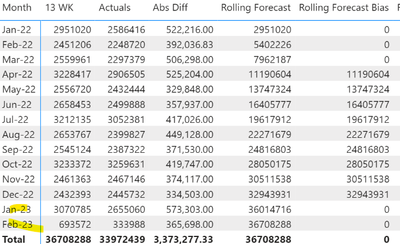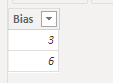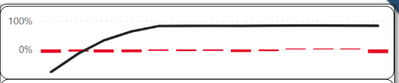cancel
Showing results for
Did you mean:Frequent Visitor

## Dax help

Hello experts,

Need help to write a new measure using DAX to calculate Rolling FC (Bias) where value gets calculated from a seleted month in the filter. Ex: bias month in this case is 3.

Bias
Filter ->  3  (coming from a filter table)

FC                Rolling FC   Rolling FC (Bias)
M1 10           10
M2 15           25
M3 20           45
M4 23           68                68
M5 15            83               83
M6 20            103             103
M7 23           126             126

Measure 1 Rolling FC
Measure 2 Rolling FC (Bias)

Any help/ direction would be highly appreciated.

Thanks,

mymsr

3 ACCEPTED SOLUTIONSFrequent Visitor

@johnyip  I managed to create the code as below. It's working fine for the year 22 but stops when  the year changes.

Rolling Forecast Bias =
IF(
MONTH(
MAX('FY Table'[Month])) >= SELECTEDVALUE('Bias table'[Bias])+1,[Rolling Forecast (13 WK)],0
)Solution Specialist

Do you have a slicer to do the filtering? If so, what is the field you have used?

By reading the screencap of your calender table, maybe you can try the following:

``Rolling Forecast Bias =IF(OR(MONTH(MAX('FY Table'[Month])) >= SELECTEDVALUE('Bias table'[Bias])+1,{{{ADD THE LOGIC THAT THE DATA YEAR IS LARGER THAN SELECTEDYEAR HERE}}}),[Rolling Forecast (13 WK)],0)``Solution Specialist

@mysmsr , perhaps you can try to see if this works.

``````Rolling Forecast Bias =
VAR VirtualTable = SUMMARIZE(ALLSELECTED(FY Table),
"Year",YEAR('FY Table'[Month])
)
VAR MinYear = VALUE(MINX(VirtualTable,[Year]))
RETURN
IF(OR(MONTH(MAX('FY Table'[Month])) >= SELECTEDVALUE('Bias table'[Bias])+1,YEAR(MAX('FY Table'[Month])) >= MinYear),[Rolling Forecast (13 WK)],0)``````

19 REPLIES 19Community Support

Hi @mysmsr ,

``````Rolling FC (Bias) =
CALCULATE (
[Rolling FC],
OFFSET (
-3,
ALLSELECTED ( 'FY Table'[Month] ),
ORDERBY ( 'FY Table'[Month], ASC )
)
)``````OFFSET

Best Regards,
Gao

Community Support Team

If there is any post helps, then please consider Accept it as the solution  to help the other members find it more quickly. If I misunderstand your needs or you still have problems on it, please feel free to let us know. Thanks a lot!

How to get your questions answered quickly --  How to provide sample data in the Power BI ForumFrequent Visitor

v-cgao-msft,

Thanks for your reply. I am not looking for offset value. I need to populate the same value in Rolling FC after few months based on the selected value from BIAS table.
I understand the current code issue. Since we are using Month() funtion it is returing month number which is independent of FY year and our condition MAX('FY Table'[Month])) >= SELECTEDVALUE('Bias table'[Bias])+1 will not work.

Regards,

RaghuSolution Specialist

Can I have a look of the structure and some sample data of Bias table?

Need to udnerstand why you wrote SELECTEDVALUE('Bias table'[Bias]), or more from your data structure to come up with the correct DAX.Frequent Visitor

Sure. Bias table is just a parameter table where I have  stored values of Bias and used it in the slicer to change the bias calculation dynamically in report.I have created a Fiscal year calendar table at month level using my fact table dates (IBP Accuracy Data).In the fact table I have my FC and Actuals stored at Monthly level.Solution Specialist

I think the most simple way is you can create another parameter table to store the value of [year], and then use the following DAX:

``Rolling Forecast Bias =IF(OR(MONTH(MAX('FY Table'[Month])) >= SELECTEDVALUE('Bias table'[Bias])+1,YEAR(MAX('FY Table'[Month])) >= SELECTEDVALUE('Year parameter table'[Year])),[Rolling Forecast (13 WK)],0)``

Or, in response to your "filter on page to get last 14 months", the following might work (you need to test if there is an error since I haven't). No parameter table / slicer of year is needed for the following:

``````Rolling Forecast Bias =
VAR VirtualTable = SUMMARIZE(ALLSELECTED(FY Table),
[Month],
"Year",MIN(YEAR('FY Table'[Month]))
)
VAR MinYear = MINX(VirtualTable,[Year])
RETURN
IF(OR(MONTH(MAX('FY Table'[Month])) >= SELECTEDVALUE('Bias table'[Bias])+1,YEAR(MAX('FY Table'[Month])) >= MinYear),[Rolling Forecast (13 WK)],0)``````Frequent Visitor

Thanks. Was trying Virtual table option.

VAR Vtable = SUMMARIZE(ALLSELECTED('FY Table'),[Month],

"Year",MIN(YEAR('FY Table'[Month]))
)
VAR MinYear = MINX(Vtable,[Year])
return
IF(OR(MONTH(MAX('FY Table'[Month]))>= SELECTEDVALUE('Bias table'[Bias])+1,
YEAR(MAX('FY Table'[Month]))>=MinYear),[Rolling Forecast (13 WK)],0)
getting error for the las column.
The MIN function only accepts a column reference as an argument.
Couldn't figureout any issue. Could you pl check..Solution Specialist

@mysmsr , perhaps you can try to see if this works.

``````Rolling Forecast Bias =
VAR VirtualTable = SUMMARIZE(ALLSELECTED(FY Table),
"Year",YEAR('FY Table'[Month])
)
VAR MinYear = VALUE(MINX(VirtualTable,[Year]))
RETURN
IF(OR(MONTH(MAX('FY Table'[Month])) >= SELECTEDVALUE('Bias table'[Bias])+1,YEAR(MAX('FY Table'[Month])) >= MinYear),[Rolling Forecast (13 WK)],0)``````Frequent Visitor

@johnyip  Thanks that worked. I just had convert them to values to use it in my calculation. Really appreciate your help 🙏Frequent Visitor

Excellent!!!. That worked. Thanks a lot.Frequent Visitor

@johnyip  I managed to create the code as below. It's working fine for the year 22 but stops when  the year changes.

Rolling Forecast Bias =
IF(
MONTH(
MAX('FY Table'[Month])) >= SELECTEDVALUE('Bias table'[Bias])+1,[Rolling Forecast (13 WK)],0
)Solution Specialist

Do you have a slicer to do the filtering? If so, what is the field you have used?

By reading the screencap of your calender table, maybe you can try the following:

``Rolling Forecast Bias =IF(OR(MONTH(MAX('FY Table'[Month])) >= SELECTEDVALUE('Bias table'[Bias])+1,{{{ADD THE LOGIC THAT THE DATA YEAR IS LARGER THAN SELECTEDYEAR HERE}}}),[Rolling Forecast (13 WK)],0)``Frequent Visitor

Hello johnyip,

Just wandering if you can help me to fix below issue please..
I have written below dax to get last month rolling actuals which is 15598152 for ytd. It works when I use card visual. But when I place it in matrix it changes.

YTD Actuals =
CALCULATE(
[Rolling Actuals],
YEAR('FY Table'[Month]) = YEAR(MAX('FY Table'[Month])) &&
MONTH('FY Table'[Month]) = MONTH(MAX('FY Table'[Month]))
)

And hence my graph gets wrong data when I plot YTD accuracy w.r.t month.thanks.

msrSolution Specialist

cannot quite understand. what is  your expected number of YTD actuals?Frequent Visitor

Sorry. My ytd actual should be a constant value of last(max) month rolling actuals which is 15598152. This value should be the same across all months. Hence I tried to capture MAX of rolling actuals in my dax code.Solution Specialist

Don't have the sample data and cannot test that. Please see if this works.

``````YTD Actuals =
VAR MaxMonth = MAXX(ALLSELECTED('FY Table'),[Month])
VAR VirtualTable = SUMMARIZE(ALLSELECTED(FY Table),
[Month],
"Rolling Actual",[Rolling Actuals]
)
VAR Rolling_Actuals = CONCATENATEX(VirtualTable,[Rolling Actual],"|")
VAR Last_Rolling_Actual = PATHITEM(Rolling_Actuals,PATHLENGTH(Rolling_Actuals))
VAR Result = IF(MAX([Month]) <> BLANK(), Last_Rolling_Actual,BLANK())
RETURN
Result``````Frequent Visitor

I have one slicer for the BIAS value from 'Bias table'[Bias]. Other than that I have used filter on page to get last 14 months. Based on Fiscal Year table (FY table) month column.Solution Specialist

How is your FY Table look like?Frequent Visitor

Hello johnyip,

Thanks for the prompt reply. my mistake i should below screen print. Month column is in dateformat from calendar table. not sure how I can share data from this platform.Regards,

RaghuSolution Specialist

@mysmsr , from your sample, maybe your biased FC's measure is as simple as follows:

``Rolling FC (Bias) = IF(MID(MAX([FC]),2,LEN(MAX([FC])))=ALLSELECTED([Your filter]),BLANK(),[Rolling FC])``

Meanwhile, if you can provide your sample data, the answer will be more guaranteed to be correct.